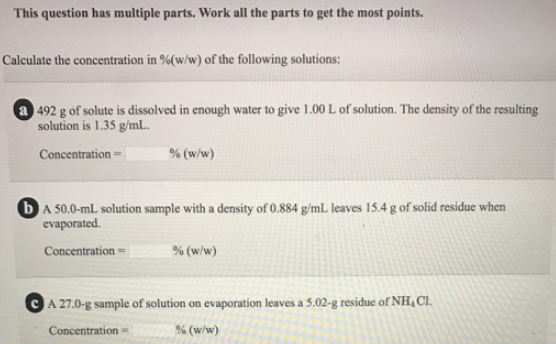# Problem: This question has multiple parts. Work on all the parts to get the most points.Calculate the concentration in %(w/w) of the following solutions: a. 492 g of solute is dissolved in enough water to give 1.00 L of solution. The density of the resulting solution is 1.35 g/mL. b. A 50.0-mL solution sample with a density of 0.884 g/mL leaves 15.4 g of solid residue when evaporated. c. A 27.0-g sample of solution on evaporation leaves a 5.02-g residue of NH 4Cl.

###### FREE Expert Solution
79% (133 ratings)###### Problem Details

This question has multiple parts. Work on all the parts to get the most points.

Calculate the concentration in %(w/w) of the following solutions:

a. 492 g of solute is dissolved in enough water to give 1.00 L of solution. The density of the resulting solution is 1.35 g/mL.

b. A 50.0-mL solution sample with a density of 0.884 g/mL leaves 15.4 g of solid residue when evaporated.

c. A 27.0-g sample of solution on evaporation leaves a 5.02-g residue of NH 4Cl.